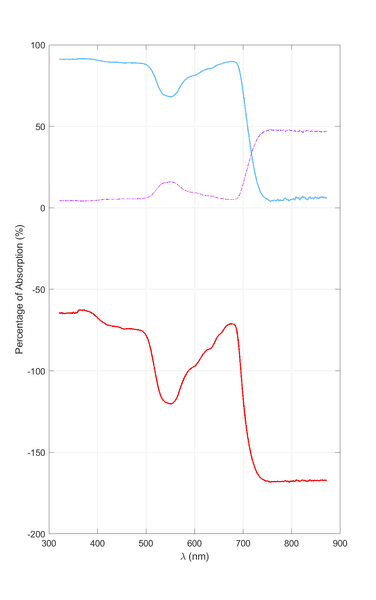# Calculation of absorption spectra

• roam
So, if you use the method that accounts for reflectance (Eq. 2), the absorption spectra will be skewed to the left because the reflectance is included in the calculation. This is usually corrected by multiplying the absorbance by 100 to make it a percentage. Regarding the negative values on the ordinate of the graph, this is usually solved by assuming a zero extinction coefficient and using Beer's law.In summary, the two methods give different results for absorbance and reflectance, and the method that accounts for reflectance (Eq. 2) usually corrects for the skewing.

#### roam

I am trying to plot the absorption curve of a given sample using some spectrophotometric data. The data that I've collected are transmittance ##T## and reflectance ##R## – the ratios of transmitted and reflected light power to incident light power. However, using two different methods I got two very different answers:

(1) When radiation impinges on a material, we know that:

$$R+A+T=1 \tag{1}$$

Therefore, if we have obtained ##R## and ##T## using a spectrophotometer, then we can simply solve for ##A## and plot the results.

(2) Most textbooks define absorbance (sometimes called optical density, OD) as:

$$A=\log_{10}\left(\frac{1}{T}\right) \tag{2}$$

If we know ##T## using a spectrophotometer then we can plot absorption using Eq. 2.

Here are my results from both methods. The top curve is calculated using Eq. 1 (dashed/dotted lines are ##T## and ##R##). The bottom curve was calculated using Eq. 2 (but the answer had to be multiplied by 100 so that it appears as a percentage).So, which is the correct method and why?Also, using the second approach we get negative values for absorption (on the ordinate of the graph). How is this usually solved in the post-processing?

Any suggestions would be greatly appreciated.

#### Attachments

•roam
I see. So to plot an absorption spectrum one has to use absorptance (Eq. 1)?

Clearly, absorptance gives the actual amount that is deposited in the material (absorption) by subtracting all the transmitted/reflected/scattered light. I know that absorbance (Eq. 2) is derived by assuming an exponential attenuation. This quantity is described (e.g. in your link) as the density of the material or the amount of attenuation the light receives. Which method would be more appropriate for comparing the absorption characteristics of two different samples?

I would always prefer to compare "internal absorbances". The internal absorbance Aint is related to the internal transmittance Tint by
Aint = - log10(Tint).
The internal transmittance is defined as the ratio of the radiant power transmitted by the sample to the incident radiant power, fully corrected for reflection losses and any window absorption. Regarding the spectroscopic nomenclature have a look at Applied Spectroscopy Spectroscopic Nomenclature - SAGE Publishing

Last edited:
•roam

## 1. What is the purpose of calculating absorption spectra?

The purpose of calculating absorption spectra is to understand the amount of light that is absorbed by a substance at different wavelengths. This can provide valuable information about the properties and behavior of the substance, such as its chemical composition and concentration.

## 2. How is the absorption spectrum calculated?

The absorption spectrum is calculated by measuring the intensity of light transmitted through a substance at different wavelengths. This data is then plotted on a graph, with the intensity on the y-axis and the wavelength on the x-axis. The resulting curve is the absorption spectrum.

## 3. What factors can affect the absorption spectrum?

The absorption spectrum can be affected by a variety of factors, such as the chemical composition of the substance, its physical state (solid, liquid, or gas), temperature, and the concentration of the substance. Additionally, the instrument used for measurement and any impurities in the sample can also impact the absorption spectrum.

## 4. What information can be obtained from the absorption spectrum?

The absorption spectrum can provide information about the chemical structure, concentration, and physical properties of a substance. It can also be used to identify unknown substances by comparing their absorption spectra to known substances.

## 5. How is the absorption spectrum used in scientific research?

The absorption spectrum is used extensively in scientific research, particularly in fields such as chemistry, biochemistry, and physics. It can be used to study the properties of substances, monitor chemical reactions, and identify unknown compounds. It is also used in various analytical techniques, such as spectrophotometry and chromatography, to quantitatively measure the amount of a substance present in a sample.